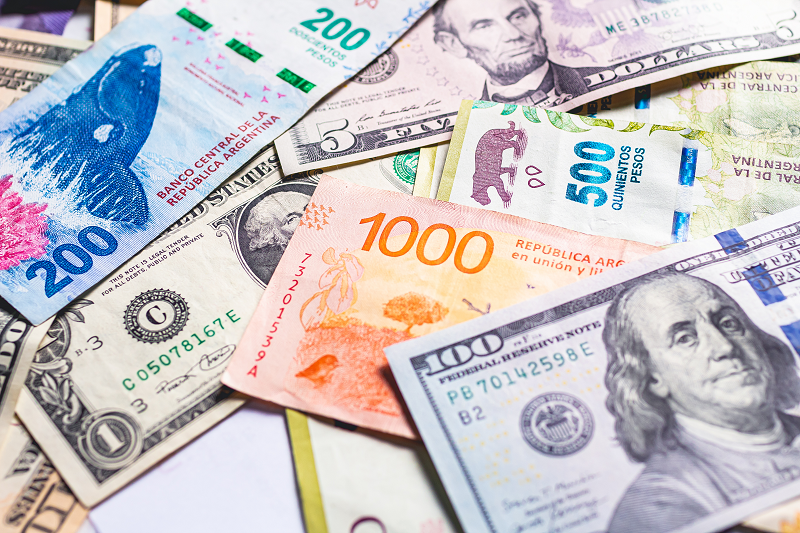Posted by & filed under Forex articles.

There are different kinds of exchange rates, and the most common of these are nominal and real exchange rates. While they both refer to the rate at which we convert one currency into another, there are significant differences between them.

Looking at their names reveals very little about what they are, how they work, and how they are determined, so we will take a look under the hood and explain what it’s all about.

### Nominal exchange rate

The nominal exchange rate (NER) is the exchange rate that we all know about. It is the exchange rate at which we swap one currency for another, or in other words, the exchange rate of one country’s currency to another – how many units of currency A are needed to purchase one unit of currency B or vice versa.

For example, if the exchange rate between the pound (GBR) and the US dollar (USD) is 1.39, then you can purchase 1.39 US dollars for £1.00. Similarly, you can buy £1.00 for \$1.39.

While that is all very straightforward, the foreign exchange market is a little more complex than that. Exchange rates can change very quickly, and there are two different nominal exchange rates – a nominal exchange rate for buying (bid) and a nominal exchange rate for selling (ask).

### Real exchange rate

Often there are significant differences in the cost of goods and services in different countries. For instance, in the United States, the price of a standard iPhone 12 is \$799, while in the UK, the price is £799.

Given the nominal exchange rate between the two currencies is £1.00 = \$1.39, it would appear substantially cheaper to buy your phone in the US. The difference would go a good way towards paying for your flight to New York.

Of course, it’s not quite as simple as that. But it’s clear that the dollar’s purchasing power is significantly higher than the pound, at least when it comes to smartphones.

Real exchange rates attempt to express the actual purchasing power of currency A relative to the true purchasing power of currency B. In other words, the real exchange rate expresses how much goods and services in one country can be exchanged for the same goods and services in another country.

To calculate the real exchange rate, we use the nominal exchange rate to express the relative prices of goods and services in the two countries in the same currency.

To calculate the real exchange rate: Let nominal exchange rate = NER and real exchange rate = RER

Then RER = NER x (price of domestic good)/(price of foreign good)

As an example, let’s use the iPhone 12 we mentioned above and assume that the NER of GBP to USD is 1:1.39

Thus the RER is 1.49 x (799/799) which is 1.49, so in this example, the NER = RER. But of course, this is an unrealistic example, as Apple has set the dollar price of the phone in the US to the same number of currency units as the pound price in the UK.

In the real world, when we wish to calculate the RER, rather than using the price of a good, we use the consumer price index. Effectively this means that we compare the price of a basket of goods from one country with the price of a basket of similar goods from another country.

Thus, we can use the RER to compare the value of exports and imports. If the RER is high, then goods from that country are relatively cheap. If the RER is low, then goods from that country are relatively expensive. This difference impacts the balance of trade. When the RER is low, we are likely to buy fewer imported goods than when the RER is high.

### Real effective exchange rate

Yet another measure of exchange rates is the real effective exchange rate (REER), which indicates the degree of alignment between currencies. The REER is the weighted average of one country’s currency to a retail price index (or basket of goods) of other major currencies. The weightings reflect the relative trade balances between countries.

While this does get quite complicated, it is an important measure that provides an insight into a country’s trading position.